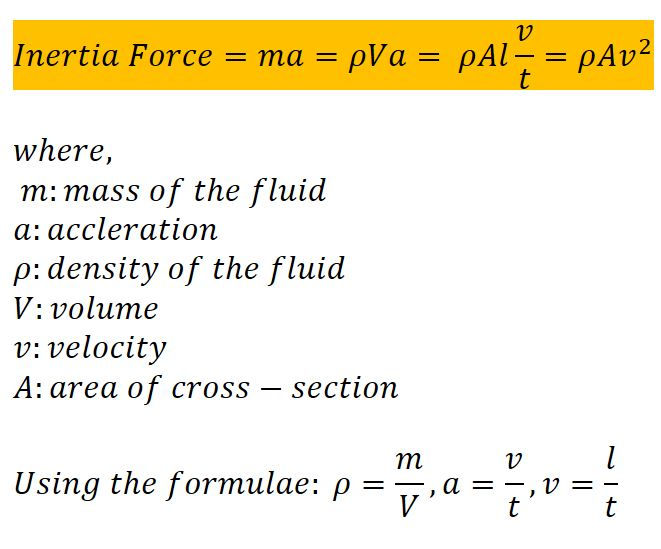top of page
Search
• Shalmali

# Boundary-Layer Formation Concept - Basics

Updated: Jul 21, 2021

Why there is relative motion between the fluid molecules? A basic introduction...Boundary
You might have seen a boundary between two countries, right? NO!!! Well me neither. But of course it exists, doesn't it?

Similarly, the fluid boundary layer also exists... but we really cannot see it. It is a concept created by humans, which does not really exist. You cannot see some "LAYER". So let's get into the concept of Boundary layer Formation.

When does this layer form?

When there is relative motion between a fluid and a solid, a boundary layer is formed. The fluid has some viscosity, and the friction between solid and fluid takes place.

Free stream velocity: It is the fluid velocity if there was no presence of solid. In short, it is the velocity in absence of any solid object or obstacle to its flow.

What is Boundary Layer?

Boundary layer can be defined as an imaginary layer of fluid, that is formed when solid and fluid are in relative motion, at a layer where velocity of fluid is equal to 99% of free stream velocity.

## Boundary-Layer Formation:

As informed before, there is no physical form of the actual boundary layer, so one has to use their imagination power to the fullest. Don't worry we will make it interesting.

Let 'v' be the free stream velocity of the air which is equal to 10m/s. Air is flowing over a still plate in X-direction as per the figure. There are four molecules namely, A, B, C, and D. They are really best friends. A is very near to the surface of the stationary plate. B is adjacent to A and far from the plate, which is followed by C and D. Let's hear their conversation.

A in conversation with B

B: Why?! Where is your velocity?

A: Actually, as the plate has zero velocity there is friction between us. As I am nearest to the surface of the stationary plate, I lost all my velocity in overcoming the friction. Yet, I am unable to move.

B: Oh! Of course, I will help you out! Here, take 8m/s.

A: Thank you B!

B in conversation with C:

C: Hey B! I overheard your conversation. You gave away a lot of velocity it seems. Here, take 5m/s of my velocity.

B: Thank you so much C!

C in conversation with D:

D: Take 0.1m/s of velocity C! Have a great day

C: Thanks!!!

Everyone helped A in this way, but the end of this story is a said one. A still cannot move and its velocity is still zero. This explains why the velocity of the molecule near the surface of the plate is zero and goes on increasing gradually.

The layer of fluid which is very close to the stationary plate is "zero". This is called a 'No-slip condition'. The fluid generally experiences 3 regions. Before that let's get to know about Reynold's Number.

### Reynold's Number:

It is the ratio of Inertia force to Viscous force. It is a dimensionless number.Inertia ForceViscous ForceReynold's Number

1. Laminar Boundary Region

This region always exists at the initial stage of the fluid flow. The adjacent layer of the fluid in this region always slide over each other. If Reynold's Number is less than 2000 it is Laminar Flow.

In the laminar region, the direction of the velocity of the molecules is the same.

2. Turbulent Boundary Region

This layer exists at the end of the fluid flow. The fluid flows randomly in any direction which causes the turbulence. Turbulence is not desired as it is very difficult to predict the motion of the fluid in the future. The modeling of the turbulent region is not easy. If Reynold's Number is greater than 4000 it is Turbulent Flow.

In the turbulent region, the direction of the velocity of the molecules is different.

3. Transient Boundary Region

A single wave changes its path and initiates the Turbulence in the fluid flow. If Reynold's Number is between 2000 and 4000, it is Transient Flow.

As the name suggests, the Transient region is just a transition region between Laminar and Turbulent flow.

### History on Boundary Layer

The boundary-layer theory began with Ludwig Prandtl's paper On the motion of a fluid with very small viscosity, which was presented at the Third International Congress of Mathematicians in August, 1904, at Heidelberg and published in the Proceedings of the Congress in the following year.

## Summary:Boundary Layer
1. When there is relative motion between a fluid and a solid a boundary layer is formed.

2. A boundary layer can be defined as an imaginary layer of fluid, that is formed when solid and fluid are in relative motion, at a layer where the velocity of the fluid is equal to 99% of free stream velocity.

3. The velocity of the molecule near the surface of the plate is zero and goes on increasing gradually.

4. There are 3 regions in a boundary layer, namely, laminar, turbulent, and transient boundary region.

5. In the laminar region, the direction of the velocity of the molecules is the same. In the turbulent region, the direction of the velocity of the molecules is different. The transient region is just a transition region between Laminar and Turbulent flow.

The boundary layer is a very vast topic and this is just a little introduction to it! Keep checking this page for further addition to this concept!